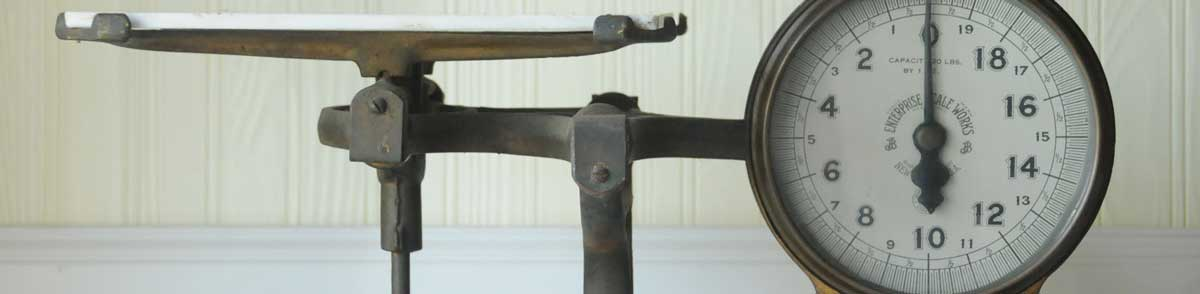# Quarts to Kilograms Conversion Calculator

Enter the volume in quarts below to calculate the weight in kilograms.

Results in Kilograms:1 qt = 0.946352946 kg
Do you want to convert kilograms to quarts?

## How to Convert Quarts to KilogramsTo convert a quart measurement to a kilogram measurement, divide the volume multiplied by the density of the ingredient or material by 1.056688.

You can use this simple formula to convert:

kilograms = quarts × ingredient density1.056688

Thus, the volume in kilograms is equal to the quarts times the density of the ingredient or material divided by 1.056688.

For example, here's how to convert 5 quarts to kilograms for an ingredient with a density of 0.7 g/mL.
5 qt = 5 × 0.71.056688 = 3.312235 kg

Quarts and kilograms are both commonly used to measure cooking ingredients.

For cooking applications, most chefs suggest measuring dry ingredients by weight rather than volume to improve accuracy in the measurements. The density of dry ingredients can vary for a variety of reasons, such as compaction.

The best way to ensure an accurate conversion is to use a scale. When a scale is not available, a calculator like the one above is a good way to estimate the volume to weight conversion.

## Quarts

The US liquid quart is a unit of fluid volume equal to one fourth of a gallon, two pints, or four cups. The liquid quart should not be confused with the dry quart (US) or the imperial quart, which are different units.

The quart is a US customary unit of volume. Quarts can be abbreviated as qt; for example, 1 quart can be written as 1 qt.

## Kilograms

The definition of the kilogram changed in 2019. One kilogram was previously equal to the mass of the platinum-iridium bar, known as the International Prototype of the Kilogram, which was stored in Sèvres, France.

The 2019 SI brochure now defines the kilogram using the Planck constant, and it is defined using the meter and second. It is roughly equal to the mass of 1,000 cubic centimeters of water.

The kilogram, or kilogramme, is the SI base unit for weight and is also a multiple of the gram. In the metric system, "kilo" is the prefix for 103. Kilograms can be abbreviated as kg; for example, 1 kilogram can be written as 1 kg.

## Quart to Kilogram Conversion Table

Quart measurements converted to kilograms for commonly used ingredients.
Volume in Quarts: Weight in Kilograms of:
Water Milk Cooking Oil All Purpose Flour Granulated Sugar
1/8 qt 0.118294 kg 0.123026 kg 0.104099 kg 0.062578 kg 0.082806 kg
1/4 qt 0.236588 kg 0.246052 kg 0.208198 kg 0.125155 kg 0.165612 kg
1/3 qt 0.315451 kg 0.328069 kg 0.277597 kg 0.166874 kg 0.220816 kg
1/2 qt 0.473176 kg 0.492104 kg 0.416395 kg 0.25031 kg 0.331224 kg
2/3 qt 0.630902 kg 0.656138 kg 0.555194 kg 0.333747 kg 0.441631 kg
3/4 qt 0.709765 kg 0.738155 kg 0.624593 kg 0.375466 kg 0.496835 kg
1 qt 0.946353 kg 0.984207 kg 0.832791 kg 0.500621 kg 0.662447 kg

## References

1. National Institute of Standards & Technology, Metric Cooking Resources, https://www.nist.gov/pml/weights-and-measures/metric-cooking-resources
2. International Bureau of Weights and Measures, The International System of Units, 9th Edition, 2019, https://www.bipm.org/documents/20126/41483022/SI-Brochure-9-EN.pdf